1. Math
2. Algebra
3. 1 find the distance between the points 2 find the...

# Question: 1 find the distance between the points 2 find the...

###### Question details

1) Find the distance between the points: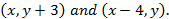2) Find the domain of the function: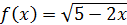3) Determine whether or not the equation represents y as function of x? Explain your answer.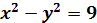4) Consider the functions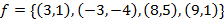and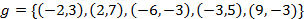. Find: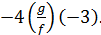###### Solution by an expert tutor